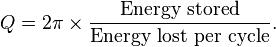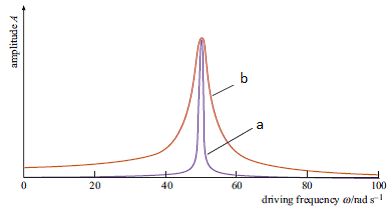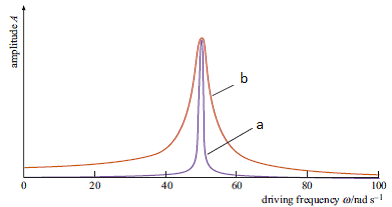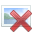# Calculating energy left in oscillator after 3 cycles

## Homework Statement

Calculate how much original energy the oscillator in will have left after three cycles.

Using Q= (ω/Δω)

I have calculated the Q factor to be 4

## Homework Equations

The answer can be obtained by using the equation

Q=2π(E/ΔE)

Where ΔE/E is the fractional energy loss per cycle of the oscillation

## The Attempt at a Solution

I have been advised that I do not need to work out the original energy, instead, work out what % or fraction of the original energy is left.

I tried using Q/2π which gave me an answer of 0.64, however the question asks over 3 cycles. Didn't know what to do after that. Fairly sure Q/2π isn't right anyway!

Any pointers would be much appreciated.

I've got a feeling you misstyped the equation Q=2π(E/ΔE). I think it should be Q=2π(ΔE/E)?

Anyways, you say that you have calculated the Q factor to be 4, so we can replace Q by four in this equation (using the "fixed" version):
Q=2π(ΔE/E) => 4=2π(ΔE/E)
By dividing by 2π on both sides, we get:
ΔE/E = 4/2π

With this, you are almost at the finish line. ΔE/E tells you how much energy the oscillator looses per cycle. If ΔE/E would turn out to be 1, this means it loses ALL its energy in one cycle. If ΔE/E would turn out to be 0, that means it would lose NO energy per cycle. In this case, it turns out to be 4/2π, which is roughly 0.63. When the oscillator begins oscillating, it has energy E. After one cycle it has lost 4/2π * E amount of its energy.

Here's the trick: After one cycle the oscillator has lost 4/2π parts of its original energy. After the second cycle, it has lost 4/2π parts of the energy that was left after the first cycle. And after the third cycle it has lost 4/2π parts of the energy that was left after the second cycle. Do you see how to set this up as a mathematical equation?

EDIT: This is incorrect, see my next post.

Last edited:
Thank you for the clear explanation, I understand what you have stated but i'm not sure what the mathematical equation would be. Please excuse my lack of knowledge, I have little or no education in this area.

If you have time, would you explain something else?

Say for example the q factor was 25, dividing this by 2pi would give an answer of 3.98. I thought the answer would need to be between 0 and 1?

Last edited:
Thank you for the clear explanation, I understand what you have stated but i'm not sure what the mathematical equation would be. Please excuse my lack of knowledge, I have little or no education in this area.

If you have time, would you explain something else?

Say for example the q factor was 25, dividing this by 2pi would give an answer of 3.98. I thought the answer would need to be between 0 and 1?

Apologies, your original equation was correct. Q is defined asYour Q value of 4 is a little strange in this case. From the equation above, we see that the minimum value of Q for an oscillator that is able to finish even a single cycle is 2π which is roughly 6.3. A Q value of 4 would mean the oscillator is critically damped and will lose all its energy before it has finished a single cycle. Could it be your Q value is wrong?This is the figure we we given. I calculated Q using ω/Δω

where Δω is the width of its peak at its halfway point

For oscillator B, I took that to be ω=50 and Δω to be (55-45)= 10

In actual fact the Q factor should be 5 but again, its less than 6.3

Have I made an error somewhere?

Last edited:
I don't immediately see an error in the way you calculated the Q value. A Q value of 5 still means that the oscillator is critically damped and will not finish even one cycle. Could you post the problem text in its entirety?

I don't immediately see an error in the way you calculated the Q value. A Q value of 5 still means that the oscillator is critically damped and will not finish even one cycle. Could you post the problem text in its entirety?

1. Taking measurements from Figure 1, determine the value of Q for each of the oscillators represented. Explain how you obtained your answer.Where Q= ω/Δω

2. Using equation 5.19 which is the rate at which the mass–spring system loses energy to its surroundings is referred
to as the Q-value for the oscillator. The Q-value is defined as:

Q=2π (E/ΔE) Eq 5.19

(Underneath the equation, this is also stated. ΔE/E is the fractional energy loss per cycle of the oscillation)

Calculate how much original energy each of the oscillators in question 1 will have left after three cycles.

My guess is that it's a trick question. If you show that oscillator B cannot possibly finish even a single cycle (without being driven by an external source), and explain your answer, it should be good. The drawing you've been given is not very detailed and quite rudimentary, giving you room for interpretation.

My guess is that it's a trick question. If you show that oscillator B cannot possibly finish even a single cycle (without being driven by an external source), and explain your answer, it should be good. The drawing you've been given is not very detailed and quite rudimentary, giving you room for interpretation.

A cheeky trick question eh? Bit naughty!

Thanks for taking the time to assist me, much appreciated.

Ryan

A cheeky trick question eh? Bit naughty!

Thanks for taking the time to assist me, much appreciated.

Ryan

Hey, no problem. Good luck!

NascentOxygen
Staff Emeritus
Remember, damping ratio ζ is related to Q as Q = 1/(2ζ).
We know a system oscillates where ζ < 0.707, which corresponds to Q > 0.707

I can't at the moment reconcile this with the 2 Pi (E/ΔE) approach.

Anyway, I'm sure this exact question was posted about a week ago. (Or else I'm a gifted chiromancer!)

Remember, damping ratio ζ is related to Q as Q = 1/(2ζ).
We know a system oscillates where ζ < 0.707, which corresponds to Q > 0.707

I can't at the moment reconcile this with the 2 Pi (E/ΔE) approach.

Anyway, I'm sure this exact question was posted about a week ago. (Or else I'm a gifted chiromancer!)

Yeah, that is peculiar. It's been some time since I last dealt with oscillators, but the way I remember it, is that the equationtells us how damped the oscillator is. When the oscillator loses all its energy in one cycle, Q = 2π, which would imply that an oscillator does not oscillate unless Q > 2π.

BvU
Homework Helper
If ##Q = {1\over 2 \zeta}## and ##Q=4##, then ##\zeta = {1\over 8}##. With the solution of the unforced equation a few lines below the Q in post #4 in wikipedia it shouldn't be too hard to find the amplitude At/A0 ratio after 3 periods.

NascentOxygen
Staff Emeritus
Certainly we can use the logarithmic decrement, but that would be evading the issue---which is why the supplied equation doesn't seem to support these other techniques.

rude man
Homework Helper
Gold Member

## Homework Statement

Calculate how much original energy the oscillator in will have left after three cycles.

Using Q= (ω/Δω)

I have calculated the Q factor to be 4

## Homework Equations

The answer can be obtained by using the equation

Q=2π(E/ΔE)

Where ΔE/E is the fractional energy loss per cycle of the oscillation

## The Attempt at a Solution

I have been advised that I do not need to work out the original energy, instead, work out what % or fraction of the original energy is left.

I tried using Q/2π which gave me an answer of 0.64, however the question asks over 3 cycles. Didn't know what to do after that. Fairly sure Q/2π isn't right anyway!

Any pointers would be much appreciated.
Your equation pertains to the steady-state solution of a resonant circuit where E is the peak energy stored in a cycle and ΔE is the energy dissipated per cycle. For this situation, E and ΔE do not change from one cycle to the next.
It is not applicable to a decaying oscillation since E and ΔE decay continuously over time. So ΔE before a particular E is larger than ΔE after the same E, and the formula is not only ambiguous but wrong.

NascentOxygen
Staff Emeritus
That sounds valid, rude man.

We should ask RM to confirm that the question supplied this information implying it was to be used in the solution:
The answer can be obtained by using the equation

Q=2π(E/ΔE)

That sounds valid, rude man.

We should ask RM to confirm that the question supplied this information implying it was to be used in the solution:

The question states:

Using equation Q=2π (E/ΔE) calculate how much original energy each of the oscillators will have left after three cycles.

Where ΔE/E is the fractional energy loss per cycle of the oscillation.

As the question asks how much original energy would be left after 3 cycles, I assumed Q could not be greater than 2π, otherwise all the energy would be dissipated before one cycle. The problem was due to the graph being difficult to read, I had measured Q to be 5.